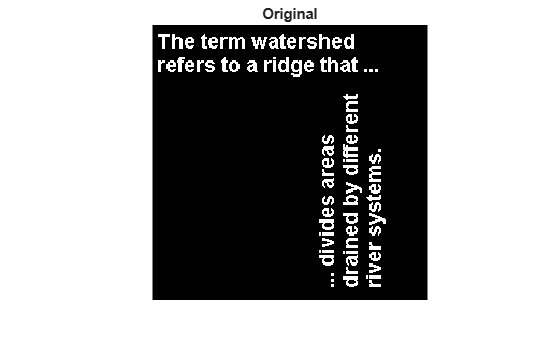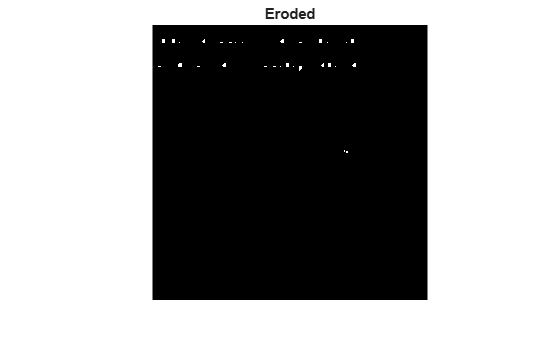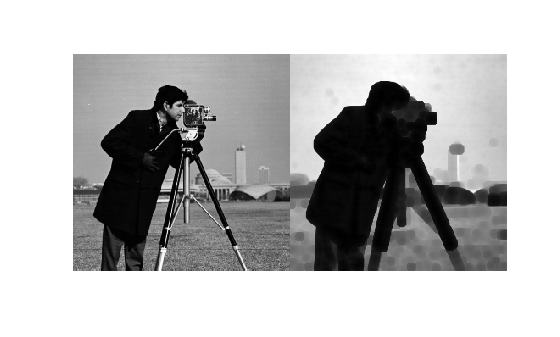# imerode

## Syntax

``J = imerode(I,SE)``
``J = imerode(I,nhood)``
``J = imerode(___,packopt,m)``
``J = imerode(___,shape)``

## Description

example

````J = imerode(I,SE)` erodes the grayscale, binary, or packed binary image `I` using the structuring element `SE`.```
````J = imerode(I,nhood)` erodes the image `I`, where `nhood` is a matrix of `0`s and `1`s that specifies the structuring element neighborhood.This syntax is equivalent to `imerode(I,strel(nhood))`. ```
````J = imerode(___,packopt,m)` specifies whether input image `I` is a packed binary image. `m` specifies the row dimension of the original unpacked image.```
````J = imerode(___,shape)` specifies the size of the output image.```

## Examples

collapse all

Read binary image into the workspace.

`originalBW = imread('text.png');`

Create a flat, line-shaped structuring element.

`se = strel('line',11,90);`

Erode the image with the structuring element.

`erodedBW = imerode(originalBW,se);`

View the original image and the eroded image.

```figure imshow(originalBW)``````figure imshow(erodedBW)```Read grayscale image into the workspace.

`originalI = imread('cameraman.tif');`

Create a nonflat `offsetstrel` object.

`se = offsetstrel('ball',5,5);`

Erode the image.

`erodedI = imerode(originalI,se);`

Display original image and eroded image.

```figure imshow(originalI)``````figure imshow(erodedI)```Create a binary volume.

```load mristack BW = mristack < 100;```

Create a cubic structuring element.

`se = strel('cube',3)`
```se = strel is a cube shaped structuring element with properties: Neighborhood: [3x3x3 logical] Dimensionality: 3 ```

Erode the volume with a cubic structuring element.

`erodedBW = imerode(BW, se);`

## Input Arguments

collapse all

Input image, specified as a grayscale image, binary image, or packed binary image of any dimension.

Data Types: `single` | `double` | `int8` | `int16` | `int32` | `uint8` | `uint16` | `uint32` | `logical`

Structuring element, specified as a scalar `strel` object or `offsetstrel` object. `SE` can also be an array of `strel` object or `offsetstrel` objects, in which case `imerode` performs multiple erosions of the input image, using each structuring element in succession.

`imerode` performs grayscale erosion for all images except images of data type `logical`. In this case, the structuring element must be flat and `imerode` performs binary erosion.

Structuring element neighborhood, specified as a matrix of `0`s and `1`s.

Example: `[0 1 0; 1 1 1; 0 1 0]`

Indicator of packed binary image, specified as one of the following.

Value

Description

`'notpacked'`

`I` is treated as a normal array.

`'ispacked'`

`I` is treated as a packed binary image as produced by `bwpack`. `I` must be a 2-D `uint32` array and `SE` must be a flat 2-D structuring element. The value of `shape` must be `'same'`.

Data Types: `char` | `string`

Row dimension of the original unpacked image, specified as a positive integer.

Data Types: `double`

Size of the output image, specified as one of the following.

Value

Description

`'same'`

The output image is the same size as the input image. If the value of `packopt` is `'ispacked'`, then `shape` must be `'same'`.

`'full'`

Compute the full erosion.

Data Types: `char` | `string`

## Output Arguments

collapse all

Eroded image, returned as a grayscale image, binary image, or packed binary image. If the input image `I` is packed binary, then `J` is also packed binary. `J` has the same data type as `I`.

collapse all

### Binary Erosion

The binary erosion of A by B, denoted A ϴ B, is defined as the set operation A ϴ B = {z|(BzA}. In other words, it is the set of pixel locations z, where the structuring element translated to location z overlaps only with foreground pixels in A.

### Grayscale Erosion

In the general form of grayscale erosion, the structuring element has a height. The grayscale erosion of A(x, y) by B(x, y) is defined as:

(A ϴ B)(x, y) = min {A(x + x′, y + y′) − B(x′, y′) | (x′, y′) ∊ DB},

DB is the domain of the structuring element B and A(x,y) is assumed to be +∞ outside the domain of the image. To create a structuring element with nonzero height values, use the syntax `strel(nhood,height)`, where `height` gives the height values and `nhood` corresponds to the structuring element domain, DB.

Most commonly, grayscale erosion is performed with a flat structuring element (B(x,y) = 0). Grayscale erosion using such a structuring element is equivalent to a local-minimum operator:

(A ϴ B)(x, y) = min {A(x + x′, y + y′) | (x′, y′) ∊ DB}.

All of the `strel` syntaxes except for `strel(nhood,height)`, `strel('arbitrary',nhood,height)`, and ```strel('ball', ...)``` produce flat structuring elements.

## Tips

• If the dimensionality of the image `I` is greater than the dimensionality of the structuring element, then the `imerode` function applies the same morphological erosion to all planes along the higher dimensions.

You can use this behavior to perform morphological erosion on RGB images. Specify a 2-D structuring element for RGB images to operate on each color channel separately.

• When you specify a structuring element neighborhood, `imerode` determines the center element of `nhood` by `floor((size(nhood)+1)/2)`.

• `imerode` automatically takes advantage of the decomposition of a structuring element object (if it exists). Also, when performing binary erosion with a structuring element object that has a decomposition, `imerode` automatically uses binary image packing to speed up the erosion .

 Gonzalez, Rafael C., Richard E. Woods, and Steven L. Eddins. Digital Image Processing Using MATLAB. Third edition. Knoxville: Gatesmark Publishing, 2020.

 Haralick, Robert M., and Linda G. Shapiro. Computer and Robot Vision. 1st ed. USA: Addison-Wesley Longman Publishing Co., Inc., 1992, pp. 158-205.

 Boomgaard, Rein van den, and Richard van Balen. “Methods for Fast Morphological Image Transforms Using Bitmapped Binary Images.” CVGIP: Graphical Models and Image Processing 54, no. 3 (May 1, 1992): 252–58. https://doi.org/10.1016/1049-9652(92)90055-3.